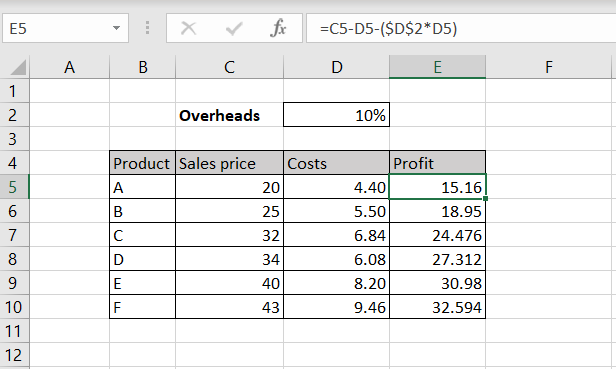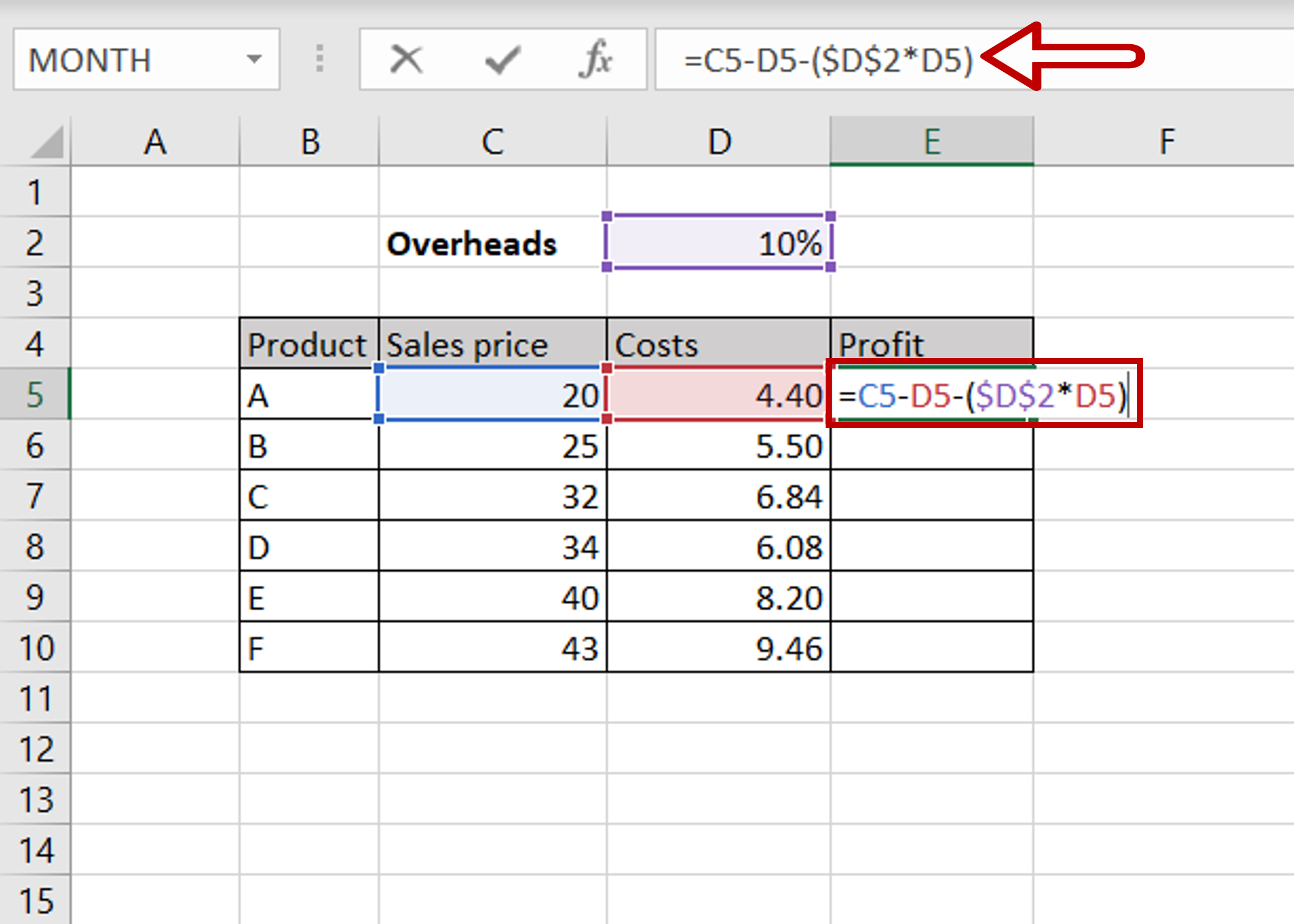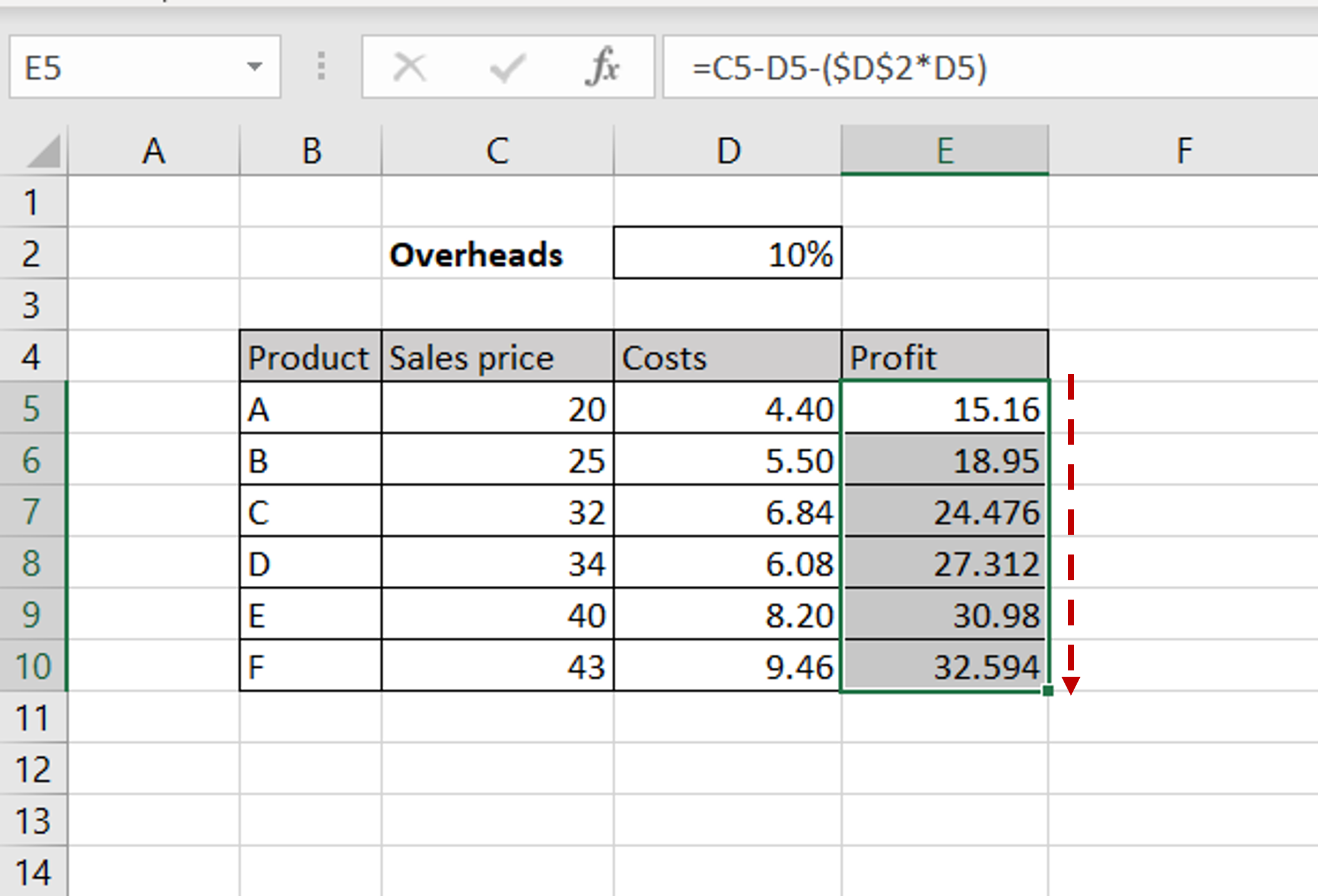# How to subtract a percentage in Excel

You can watch a video tutorial here.Excel is a great tool for performing calculations across many columns and for creating complex formulas.  Here we will see how to subtract the percentage of a value from a number.

### Step 1 – Create the formula– The objective is to find the profit by subtracting Costs and Overheads from the Sales price
– Select the first cell in the ‘Profit’ column
– Type in the formula using cell references:
= Sales price – Costs – (\$Overheads * Costs)
Make the ‘Overheads’ constant by selecting the cell reference and pressing F4
– Press Enter

### Step 2 – Copy the formula– Using the fill handle from the first cell, drag the formula to the remaining cells
OR
a) Select the cell with the formula and press Ctrl+C or choose Copy from the context menu (right-click)
b) Select the rest of the cells in the column and press Ctrl+V or choose Paste from the context menu (right-click)